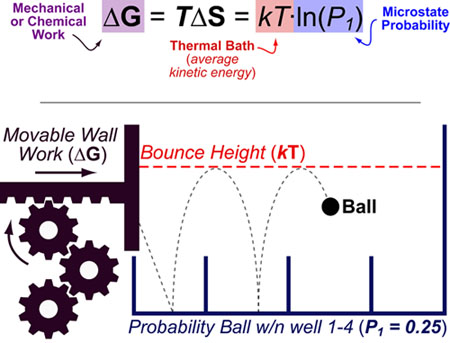# What is Entropy??Entropy (S) can be best understood as “the effect of probability on a physical or chemical processes”. This relationship is famously described by the Boltzmann entropy formula which relates the probability of a particular state (P1) to the chemical or mechanical work (ΔG) required to obtain that state.
Entropy changes(ΔS), are not probabilities per se but rather a conceptual bridge between probability and energy. In this equation, k is the Boltzmann constant, T is temperature, P is the probability of the considered state, ΔS is the entropy change and ΔG is the free energy change.

The Boltzmann formula can be intuitively understood by considering a bouncing ball in a room with movable walls: The probability that the ball will land in Box 1 is P1 and the energy required to force the ball into Box 1 is ΔG. kT represents the average kinetic energy of the ball and can be thought of as the “bouncing height” of the ball. This kinetic energy term affects both the probabilities of accessible states (as the ball must have enough energy to surpass barriers between boxes) and the energy necessary to change those probabilities (ΔG).

### REFERENCES:

1. Philips, R.; Kondev, J., Theriot, J. Physical Biology of the Cell Garland Science 2009
2. McQuarrie, D. A.; Simon, J. D. Physical Chemistry: A Molecular Approach., University Science Books, 1999